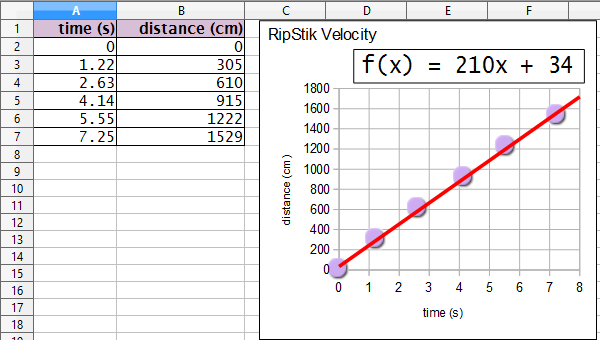psd3 024 ☈ 29 August 2014 Name:

$\text{slope}=\frac{\left({y}_{2}-{y}_{1}\right)}{\left({x}_{2}-{x}_{1}\right)}$
$ѵ=\frac{\Delta d}{\Delta t}$
distance d = velocity ѵ × time t

1. The graph shows the time versus distance data gathered for four different ball speeds in laboratory 02.
1. __________ _____ Determine the velocity ѵ of ball A.
2. __________ _____ Determine the velocity ѵ of ball B.
3. __________ _____ Determine the velocity ѵ of ball C.
4. __________ _____ Determine the velocity ѵ of ball D.
2. __________ _____ Calculate the velocity ѵ of a ball that rolls 2424 centimeters in six seconds.
3. __________ _____ Calculate the distance a ball with a velocity ѵ of 777 cm/s will roll in six seconds.
4. __________ _____ Calculate the time for a ball with a velocity ѵ of 243 cm/s to roll 729 centimeters.
5. For the following RipStik velocity data and chart:1. __________ _____ Determine the velocity ѵ of the RipStik.
2. __________ _____ If the RipStik continued at that velocity for 60 seconds, how many centimeters would the RipStik travel?
3. __________ _____ If the RipStik continued at that velocity for 1819 centimeters, how many seconds would the RipsStik travel?
6. The five graphs seen below plot time versus distance for a rolling ball. Time in seconds is on the x-axis. Distance in centimeters is on the y-axis.
1. Explain what is happening with the speed of ball A.
2. Explain what is happening with the speed of ball B.
3. Explain what is happening with the speed of ball C.
4. Explain what is happening with the speed of ball D.
5. Explain what is happening with the speed of ball E.